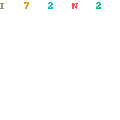Methods of Algebraic Geometry in Control Theory: Part II: Multivariable Linear Systems and Projective Algebraic GeometryMethods of Algebraic Geometry in Control Theory: Part II: Multivariable Linear Systems and Projective Algebraic Geometry by Peter Falb
English | PDF | 2018 | 384 Pages | ISBN : 3319965735 | 24.56 MB

“An introduction to the ideas of algebraic geometry in the motivated context of system theory.” This describes this two volume work which has been specifically written to serve the needs of researchers and students of systems, control, and applied mathematics.

Without sacrificing mathematical rigor, the author makes the basic ideas of algebraic geometry accessible to engineers and applied scientists. The emphasis is on constructive methods and clarity rather than on abstraction. While familiarity with Part I is helpful, it is not essential, since a considerable amount of relevant material is included here.
Part I, Scalar Linear Systems and Affine Algebraic Geometry, contains a clear presentation, with an applied flavor , of the core ideas in the algebra-geometric treatment of scalar linear system theory. Part II extends the theory to multivariable systems. After delineating limitations of the scalar theory through carefully chosen examples, the author introduces seven representations of a multivariable linear system and establishes the major results of the underlying theory. Of key importance is a clear, detailed analysis of the structure of the space of linear systems including the full set of equations defining the space. Key topics also covered are the Geometric Quotient Theorem and a highly geometric analysis of both state and output feedback.
Prerequisites are the basics of linear algebra, some simple topological notions, the elementary properties of groups, rings, and fields, and a basic course in linear systems. Exercises, which are an integral part of the exposition throughout, combined with an index and extensive bibliography of related literature make this a valuable classroom tool or good self-study resource. The present, softcover reprint is designed to make this classic textbook available to a wider audience.
“The exposition is extremely clear. In order to motivate the general theory, the author presents a number of examples of two or three input-, two-output systems in detail. I highly recommend this excellent book to all those interested in the interplay between control theory and algebraic geometry.” —Publicationes Mathematicae, Debrecen
“This book is the multivariable counterpart of Methods of Algebraic Geometry in Control Theory, Part I…. In the first volume the simpler single-input–single-output time-invariant linear systems were considered and the corresponding simpler affine algebraic geometry was used as the required prerequisite. Obviously, multivariable systems are more difficult and consequently the algebraic results are deeper and less transparent, but essential in the understanding of linear control theory…. Each chapter contains illustrative examples throughout and terminates with some exercises for further study.” —Mathematical Reviews